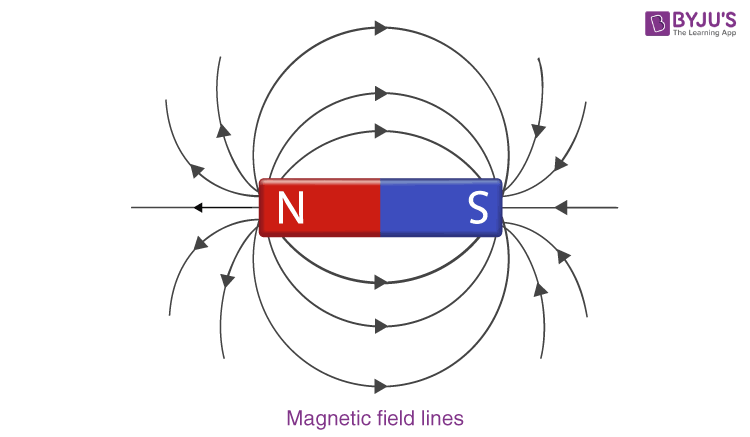# Magnetic Dipole Moment

A magnetic moment is a quantity that represents the magnetic strength and orientation of a magnet or any other object that produces a magnetic field. More precisely, a magnetic moment refers to a magnetic dipole moment, the component of the magnetic moment that can be represented by a magnetic dipole. A magnetic dipole is a magnetic north pole and a magnetic south pole separated by a small distance.

Magnetic dipole moments have dimensions of current times area or energy divided by magnetic flux density. The unit for dipole moment in metre–kilogram– second–ampere is ampere-square metre. The unit in centimetre–gram–second unit system, is the erg (unit of energy) per gauss (unit of magnetic flux density). One thousand ergs per gauss equal to one ampere-square metre.

Following is the table explaining other dipole related concepts

## Derivation of Magnetic Dipole Moment Formula

Magnetic Dipole Moment- The magnetic field, B due to a current loop carrying a current i of radius, R at a distance l along its axis is given by:

$$\begin{array}{l} B = \frac {μ_0 i R^2}{2(R^2~+~l^2 )^{\frac32}}\end{array}$$

Now if we consider a point very far from the current loop such that l>>R, then we can approximate the field as:

$$\begin{array}{l} B = \frac{μ_0 i R^2}{2l^3 \left( \left(\frac{R}{l} \right)^2~+~1\right)^\frac 32 } \approx \frac {μ_0 i R^2}{2l^3} \equiv \frac {μ_0}{4π} \frac{2i (πR^2)}{l^3}\end{array}$$

Now, the area of the loop, A is

$$\begin{array}{l}A = πR^2 \end{array}$$

Thus, the magnetic field can be written as

$$\begin{array}{l} B = \frac {μ_0}{4π} \frac {2i A}{l^3} = \frac {μ_0}{4π } \frac {2μ}{l^3} \end{array}$$

We can write this new quantity μ as a vector that points along the magnetic field, so that

$$\begin{array}{l} \overrightarrow{B} = \frac {μ_0}{4π} \frac {2 \overrightarrow{μ}}{l^3}\end{array}$$

Notice the astounding similarity to the  electric dipole field:

$$\begin{array}{l} \overrightarrow{E}=\frac {1}{4 \pi \epsilon_0} \frac {2 \overrightarrow {p}}{r^3}\end{array}$$

Unlike electric fields, magnetic fields do not have ‘charge’ ‘counterparts. In other words there are no sources or sinks of magnetic fields, there can only be a dipole. Anything that can produce a magnetic field comes with both a source and a sink i.e. there is both a north pole and a South Pole. In many ways, the magnetic dipole is the fundamental unit that can produce a magnetic field.

Most elementary particles behave intrinsically as magnetic dipoles. For example, the electron itself behaves as a magnetic dipole and has a spin magnetic dipole moment. This magnetic moment is intrinsic as the electron has neither an area A (it is a point object) nor does it spin around itself, but is fundamental to the nature of the electron’s existence.

We can generalize the magnetic moment for ‘N’ turns of the wire loop as

μ = NiA

The magnetic field lines of a current loop look similar to that of an idealized electric dipole:If you have ever broken a magnet into two parts, you would have found that each piece forms a new magnet. The new pieces also contain a north and a South Pole. You just can’t seem to be able to obtain just a north pole. Can you find the answer in this article?

### What Is the Magnetic Dipole Moment of a Revolving Electron?

The magnetic dipole moment of a revolving electron is given as:

The current of an electron revolving around a heavy nucleus is given as:

$$\begin{array}{l}I = \frac{e}{T}=\frac{e}{\frac{2\pi R}{V}}=\frac{eV}{2\pi R}\end{array}$$

The magnetic moment associated to the current of an electron revolving is given as:

$$\begin{array}{l}\mu _{l}=I_{A}=\frac{eV}{2\pi R}\times \pi R^{2}=\frac{eVR}{2}\end{array}$$

Substituting the angular momentum of the revolving electron we get,

l = mVR

$$\begin{array}{l}VR=\frac{l}{m}\end{array}$$

Therefore,

$$\begin{array}{l}\mu _{l}=\frac{el}{2m}\end{array}$$
$$\begin{array}{l}\frac{\mu_{l}}{l}=\frac{e}{2m}\end{array}$$

The above equation is known as Gyromagnetic ratio.

### How Does an Atom Behave as a Magnetic Dipole?

The electrons in an atom revolve around the nucleus in a closed orbit. The orbit around the nucleus is equivalent to a current loop as the electrons are charged particles. The electrons revolve in anticlockwise while the current revolves in the clockwise direction. This movement of electrons creates a south pole and north pole resulting in atom’s behavior as a magnetic dipole.

### What Is Magnetic Dipole Moment of a Current Loop?

The magnetic dipole moment of a current loop carrying current I with area A is given as the magnitude of m:

$$\begin{array}{l}\left | m \right | = IA\end{array}$$

The direction of the magnetic dipole moment is perpendicular to the plane of the current loop.

## Class 12 Physics magnetism and matter chapter analysisStay tuned to BYJU’S and Fall in Love with Learning!

Test your knowledge on Magnetic Dipole Moment